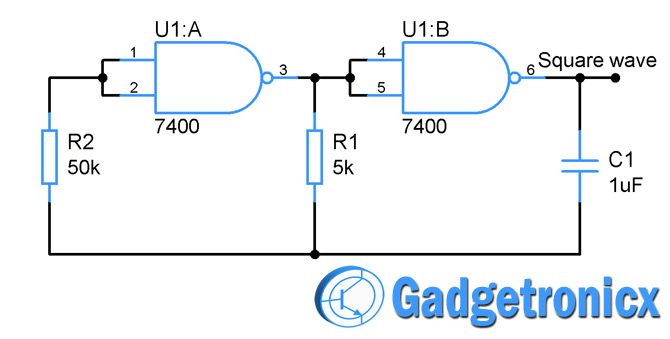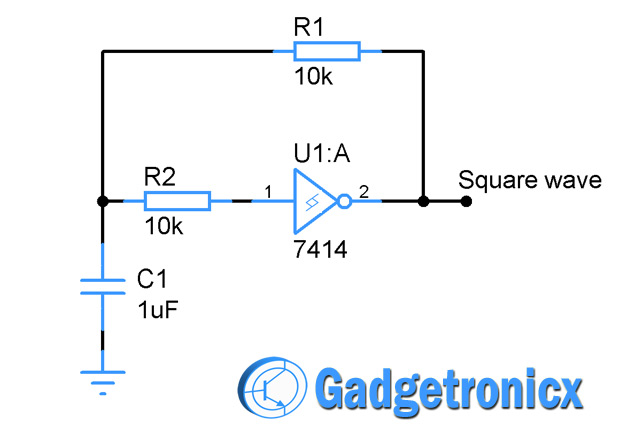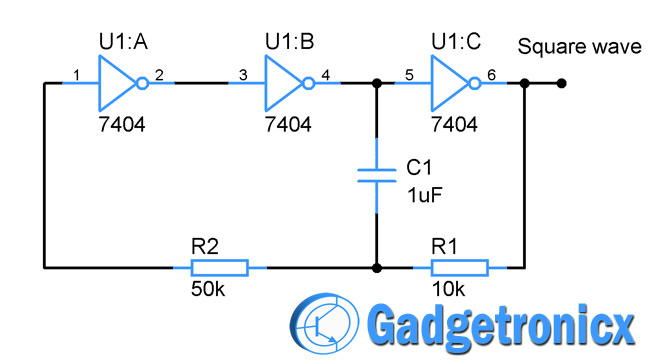Browse over 10,000 Electronics Projects

# Square wave generator using Logic gatesSquare waves are extensively used in many digital circuits. Many combinational logic circuits require this wave to operate. Here are few methods you can generate simple square wave using NAND, Inverter and Schmitt Trigger gates. These kind of square wave generator fit perfectly for simple oscillator applications with minimum effort required to build.

## SQUARE WAVE GENERATOR USING NAND GATE:You can generate square waves using two nand gates connecting together. I have used 7400 IC which is a quad two input NAND gate IC. We all know that NAND gates output will be high for input states 00, 10 and 01 and low for input 11. Here both the input pins are connected together following the same logic. The RC circuit R1C1 forms the important timing element in this circuit.

The R1C1 network is controlled by the first NAND gate U1:A and this is fed back to the input of U1:A which operates in loop to generate square waves. When the low input is fed into U1:A high output is exhibited from it. This starts charging the capacitor through R1 when the Capacitor Voltage V reaches the input threshold U1:A the output of U1:A switches to low 0. The capacitor starts discharging and thus repeats the same procedure over and over again forming square waves.

The frequency of the square wave is given by f = 1 / 2.2 R1C1

## SQUARE WAVE GENERATOR USING SCHMITT TRIGGER INVERTER:This square wave generator is made out of 7414 a Hex Schmitt trigger inverter IC. We knew that Schmitt trigger operates with the threshold level of the input unlike digital inverter gates. The timing of the circuit is governed by the R1C1 RC circuit. The output of the inverter to fed into the input providing positive feedback to it.

When the input of the inverter gate is  less than the minimum threshold of the trigger the output state will be high. This makes the capacitor to charge through the resistor R1. When the capacitor voltage reaches the maximum threshold of the trigger output changes to low state. This discharges the capacitor and the drop in voltage forces the cycle to repeat again.

The frequency of the generated square wave is given by the formula f = 1/1.2 R1C1

## SQUARE WAVE GENERATOR USING NOT GATE:This follows the similar principle of the other two circuits and built around 7404 a hex inverter IC chip. The timing element is again controlled by the RC network R1C1 and R2 is meant to provide feedback input to the U1:A inverter.

When the input at U1:A is low the output of the U1:C will be in high state and this makes the capacitor to charge through R1. When the capacitor voltage C1 is high to trigger the U1:A its output state changes. This causes the capacitor to discharge and again when the voltage drops the U1:A output changes its state and the whole procedure will repeat in loop to generate square waves.

The frequency of the above circuit can be given by the formula f = 1/ 1.1 R1C1

### NOTE:

• The power and ground pins are omitted in all the circuit diagrams to make it simple.
• These kind of square wave generators was easy to build and have some limitations such as maintaining the duty cycle for a long period of time, so fit goof for simple applications.

Top